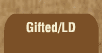Mathematics and Dyslexia

Tips for Learning the Times Tables

Tools for the Times Tables & Workbook

Home > Math > times tables practice > zeroes and ones times tables

## Times Tables Quiz: Stage One, Level Three

Cumulative Quiz: Zeroes and Ones

Answer these questions; when you're done, click "grade me!" and you'll find out how many you got right.

Use the tab key to go to the next blank. Use "Shift/Tab" to go back. Or, use your trusty mouse.

1. 8 x 1=
2. 3 x 0=
3. 1 x 1=
4. 0 x 9=
5. 0 x 1=
6. 0 x 7=
7. 8 x 1=
8. 5 x 0=
9. 1 x 4=
10. 24 x 1=
11. 0 x 8=
12. 4002 x 1=
13. 5 x 1=
14. 7 x 0=
15. 2 x 1=
16. 10 x 1=
17. 0 x 10=
18. 0 x 0=
19. 3 x 1=
20. 6 x 1=
21. 1 x 0=
22. 0 x 5=
23. w x 1=
24. pickle x 0=
25. 1 x bassoon=
26. Bonus! 7 x 6=